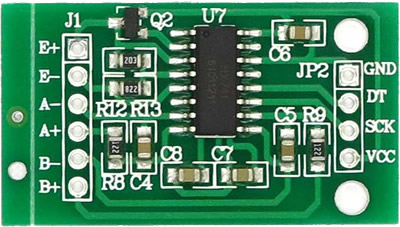# UPM

The UPM API is a high level sensor library for IoT devices using MRAA. See examples here. Back to index page.
SparkFun sensor images provided under CC BY-NC-SA-3.0.

# HX711 Class

Module: hx711

HX711 is a precision 24-bit analog-to-digital converter (ADC) designed for weight scales and industrial control applications to interface directly with a bridge sensor. This module was tested on the Intel(R) Galileo Gen 2 board.## Methods

### `HX711`

(
• `data`
• `sck`
• `gain`
)
Number

HX711 constructor

#### Parameters:

• `data` Number

Defines the data pin

• `sck` Number

Defines the clock pin

• `gain` Number

Defines the gain factor Valid values are 128 or 64 for channel A; channel B works with a 32-gain factor only

Number:

() Number

Number:

### `setGain`

(
• `gain`
)

Sets the gain factor; takes effect only after a call to read() channel A can be set for a 128 or 64 gain; channel B has a fixed 32-gain factor depending on the parameter; the channel is also set to either A or B

#### Parameters:

• `gain` Number

Defines the gain factor

### `readAverage`

(
• `times`
)
Number

#### Parameters:

• `times` Number

Defines how many reading to do

Number:

### `getValue`

(
• `times`
)
Number

#### Parameters:

• `times` Number

Defines how many readings to do

#### Returns:

Number:

Current value without the tare weight

### `getUnits`

(
• `times`
)
Number

Returns getValue() divided by SCALE

#### Parameters:

• `times` Number

Defines how many readings to do

#### Returns:

Number:

Raw value divided by a value obtained via calibration

### `tare`

(
• `times`
)

Sets the OFFSET value for the tare weight

#### Parameters:

• `times` Number

Defines how many times to read the tare value

### `setScale`

(
• `scale`
)

Sets the SCALE value This value is used to convert the raw data to human-readable data (measurement units)

#### Parameters:

• `scale` Number

Value obtained via calibration